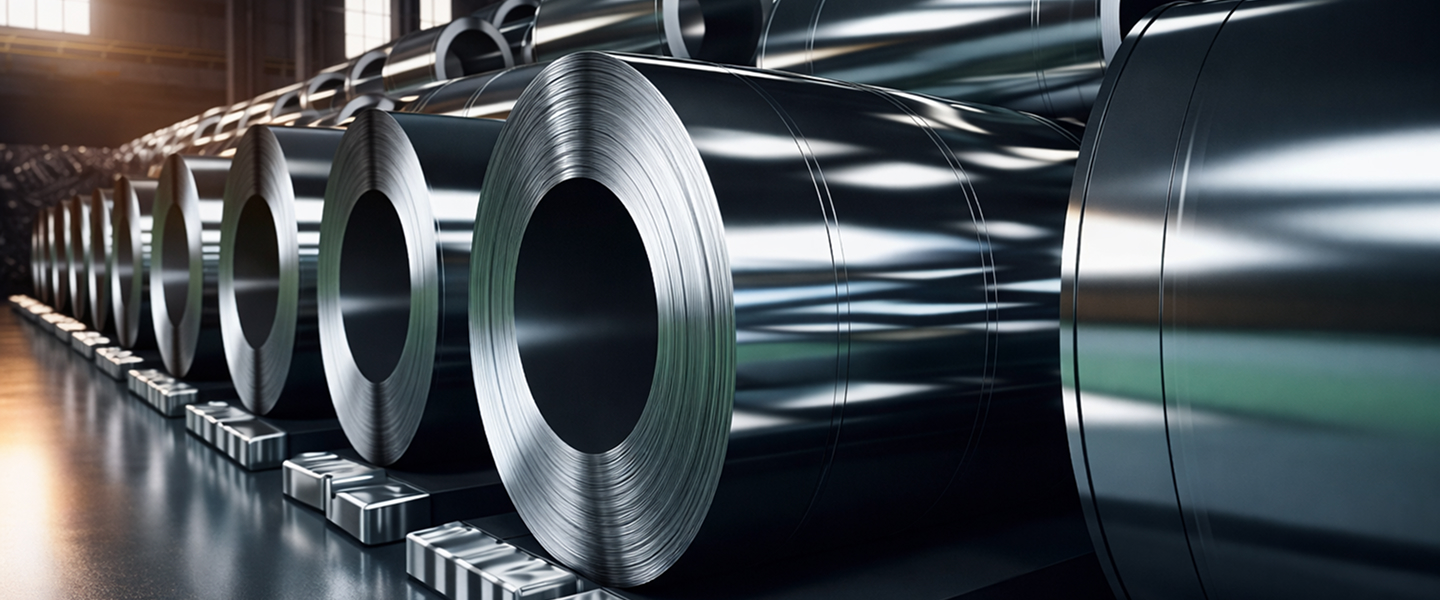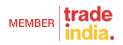Calculation Chart
 Formula of Weight: Weight of Stainless Steel Pipes & Tubes OD (mm)-W.T. (mm) x W.T. (mm) x 0.02466 = Kg.per Mtr. Sheet Width Required for rolled and welded pipes O.D. (mm) - THK (mm) x 3.14 = Sheet Width Weight of Stainless Steel Sheets Length (mtr.) x Wdth (mtr.) x Thk (mm) x 8 = Kg Per Sheet Weight of Stainless Steel Circle & Blanks OD(mm) x OD (mm) x Thk (mm) / 160 / 1000 = Kg Per Pcs. Weight of Stainless Steel Rounds Dia. (mm) x dia.(mm) x 0.00623 = PerMtr. Weight of Stainless Steel Hexagonal Rods Dia. (mm) x Dia. (mm) x 0.00679=Per Mtr. Weight of stainless Steel Square Bars Dia. (mm) x Dia. (mm) x 0.00787=Per Mtr. Weight of Carbon Steel Sheets & Plates Length (mtr.) x Wdth (mtr.) x Thk (mm) x 7.85 = Kg Per Sheet Weight of Copper Pipes OD (mm) - W.T. (mm) x W.T.(mm) x 0.0256 = Kg.per Mtr. Weight of Lead Pipes(appro.) OD (mm) - W.T. (mm) x W.T. (mm) x 0.0345 = per Mtr. Weight of Lead Sheets (Approx) Length (mtr.) x Width (mtr.) x Thk (mm) x 11.2 = Kg Per Sheet Weight of Alluminium Pipes (Approx) OD (mm) - W.T. (mm) x W.T. (mm) x 0.0082 = Kg per Mtr. Weight of Alluminium Sheets (Approx) Length (mtr.) x Width (mtr.) x Thk (mm) x 2.66 = Kg Per Sheet Tensile Strength Conversion Table: Kg/mm2 x 9.81 = N/mm2=MPa PSi x 0.0007 = Kg/mm2 KSi x 1000 = PSi Kg/mm2 x 1.422 = KSiRegn. No: QAIC/IN/512-ANIKO STEEL AND ENGINEERING LLP All Rights Reserved.(Terms of Use)Developed and Managed by Infocom Network Private Limited.